[Introduction]  [Signal arithmetic]  [Signals and noise]   [Smoothing]   [Differentiation]  [Peak Sharpening]  [Harmonic analysis]   [Fourier convolution]  [Fourier deconvolution]  [Fourier filter]  [Wavelets]   [Peak area measurement]  [Linear Least Squares]  [Multicomponent Spectroscopy]  [Iterative Curve Fitting]  [Hyperlinear quantitative absorption spectrophotometry] [Appendix and Case Studies]  [Peak Finding and Measurement]  [iPeak]   [iSignal]  [Peak Fitters]   [iFilter]  [iPower]  [List of downloadable software]  [Interactive tools]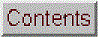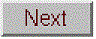## Integration and peak area measurement

The symbolic integration of functions and the calculation of definite integrals are topics that are introduced in elementary Calculus courses. The numerical integration of digitized signals finds application in analytical signal processing mainly as a method for measuring the areas under the curves of peak-type signals.

Peak area measurements are very important in chromatography, a class of chemical measurement techniques in which a mixture of components is made to flow through a chemically-prepared tube or layer that allows some of the components in the mixture to travel faster than others, followed by a device called a detector that measures and records the components after separation. Ideally, the components are sufficiently separated so that each one forms a distinct peak in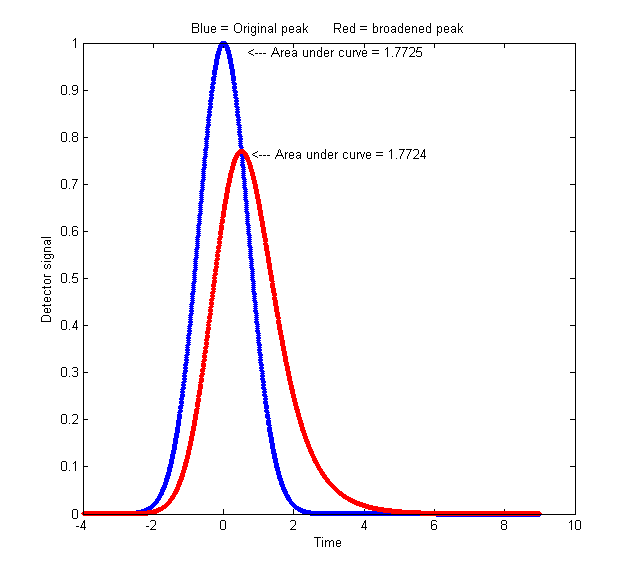the detector signal as a function of time. The magnitude of the peaks are calibrated to the concentration of that component by measuring the peaks obtained from "standard solutions" of known concentration. In chromatography it is common to measure the area under the detector peaks rather than the height of the peaks, because peak area is less sensitive to the influence of peak broadening (dispersion) mechanisms that cause the molecules of a specific substance to be be diluted and spread out rather than being concentrated on one "plug" of material as it travels down the column. These dispersion effects, which arise from many sources, cause chromatographic peaks to become shorter, broader, and in some cases more unsymmetrical, but they have little effect on the total area under the peak, as long as the total number of molecules remains the same. If the detector response is linear with respect to the concentration of the material, the peak area remains proportional to the total quantity of substance passing into the detector, even though the peak height is smaller. A graphical example is shown on the left (Matlab/Octave code), which plots detector signal vs time, where the blue curve represents the original signal and the red curve shows the effect of broadening by dispersion effects. The peak height is lower and the width is greater, but the area under the curve is almost exactly the same. If the extent of broadening changes between the time that the standards are run and the time that the unknown samples are run, then peak area measurements will be more accurate and reliable than peak height measurements. (Peak height will be proportional to the quantity of material only if the peak width and shape are constant). Another example with greater broadening: (script and graphic).

Peak area measurements are occasionally used also in spectroscopy, for example in flow injection analysis and in graphite furnace atomic absorption (reference 87). In that application, calibration curves based on area measurements are more linear than peak height measurements because most of the area of a peak is measured when the transient absorbance is less than maximum and where Beer's Law is more strictly obeyed.

On the other hand, peak height measurements are simpler to make and are less prone to interference by neighboring, overlapping peaks. And a further disadvantage of peak area measurement is that the peak start and stop points must be determined, which may be difficult especially if the multiple peaks overlap. In principle, curve fitting can measure the areas of peaks even then they overlap, but that requires that the shapes of the peaks be known at least approximately  (however, see PeakShapeAnalyticalCurve.m described in the Appendix).

Chromatographic peaks are often described as a Gaussian function or as a convolution of a Gaussian with an exponential function. A detailed quantitative comparison of peak height and peak area measurement is given in Appendix L: Why measure peak area rather than peak height? (In spectroscopy, other broadening mechanisms are often encountered, such as Doppler broadening caused by thermal motion, which results in a Gaussian broadening function).

Before computers, there were several methods used to compute peak areas that sound strange by today's standards:
(a) plot the signal on a paper chart, cut out the peak with scissors, then weigh the cut out piece on a micro-balance compared to a square section of known area;
(b) count the grid squares under a curve recorded on gridded graph paper,
(c) use a mechanical ball-and-disk integrator,
(d) use geometry to compute the area under a triangle constructed with its sides tangent to the sides of the peak, or
(e) compute the cumulative sum of the signal magnitude and measure the heights of the resulting steps (see figure below).
But now that computing power is built into or connected to every measuring instrument, more accurate and convenient digital methods can be employed. However it is measured, the units of peak area are the product of the x and y units. Thus, in a chromatogram where the x is time in minutes and y is volts, the area is in volts-minute. In absorption spectrum where the x is nm (nanometers) and y is absorbance, the area has the units of absorbance-nm. Because of this, the numerical magnitude of peak area will always be different from that of the peak height. If you are performing a quantitative analysis of unknown samples by means of a calibration curve, you must use the same method of measurement for both the standards and the samples, even if the measurements are inaccurate, as long as the error is the same for all standards and samples (which is why an approximate method like triangle construction works better than expected for quantitative analysis).

The best method for calculating the area under a peak depends whether the peak is isolated or overlapped with other peaks or superimposed on a non-zero baseline or not. For an isolated peak, Yuri Kalambet (reference 71) has shown that the trapezoidal rule area is efficient estimate of full peak area with extraordinary low error, whereas Simpson's rule is less efficient in full area integration. For a Gaussian peak, the trapezoidal rule requires 0.62 points per standard deviation (or 2.5 points within the 4*sigma basewidth) to achieve an integration error of only 0.1%.  This is demonstrated by a digital simulation of the effect of sampling rate (data density) on the accuracy of  peak area measurements for single isolated sparsely sampled Gaussian peaks (below left).

Computing the cumulative sum will convert a series of peaks into a series of steps, the height of each of which is proportional to the area under that peak (above, right). But this works well only if the peaks are well separated from each other and if the baseline is zero. This is a commonly used method in proton NMR spectroscopy, where the area under each peak or multiplet is proportional to the number of equivalent hydrogen atoms responsible for that peak.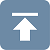The classical way to handle the overlapping peak problem is to draw two vertical lines from the left and right bounds of the peak down to the x-axis and then to measure the total area bounded by the signal curve, the x-axis (y=0 line), and the two vertical lines, shown the the shaded area in the figure on the left, below. This is often called the perpendicular drop method; it's an easy task for a computer, although tedious to do by hand. The left and right bounds of the peak are usually taken as the valleys (minima) between the peaks or as the point half-way between the peak center and the centers of the peaks to the left and right. The basic assumption is that the area missed by cutting off the feet of one peak is made up for by including the feet of the adjacent peak. This is accurate only of the peaks are symmetrical, not too overlapped, and equal in height and in width. In addition, the baseline must be zero; any extraneous background signal must be subtracted before measurement. Using this method it is possible to estimate the area of the second peak in the example below to an accuracy of about 0.3%, but the last two peaks give errors greater than 4%. As a rough rule, the valley between the peaks must be quite low, perhaps a quarter or a fifth of the adjacent peak height, for this method to be acceptable. Even so, this method is widely used because there is no simple alternative. If there is no valley between the peaks you need to measure, it's possible to apply peak sharpening techniques to narrow the peaks and deepen the valley before the perpendicular drop measurement; see PeakSharpeningAreaMeasurementDemo.xlsm (screen image). Moreover, asymmetrical peaks that are the result of exponential broadening can be narrowed and symmetricalized by the weighted addition of its first derivative, making the perpendicular drop area measurements much more accurate. In both cases, it may be necessary to set the strength of sharpening higher than previously recommended, if it that is the only way to form a valley between peaks whose areas you want to measure.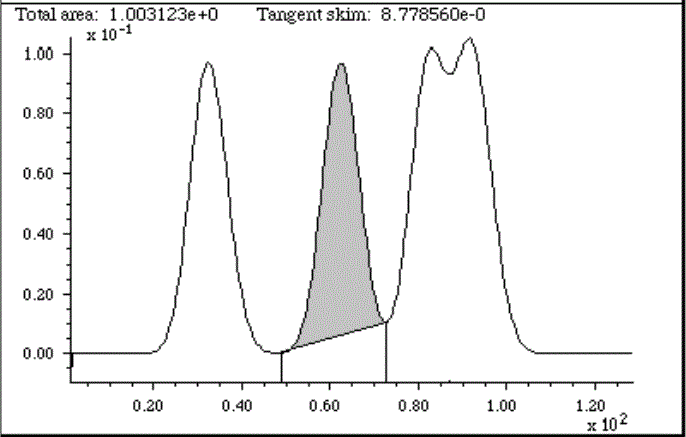Peak area measurement for overlapping peaks, using the perpendicular drop method (left, shaded area)
and tangent skim method (right, shaded area).

In the case where a single peak is superimposed on a straight or broadly curved baseline, you might use the tangent skim method, which measures the area between the curve and a linear baseline drawn across the bottom of the peak (e.g. the shaded area in the figure on the right, above). In general, the hardest part of the problem and the greatest source of uncertainty is determining the shape of the baseline under the peaks and determining when each peaks begins and ends. Once those are determined, you subtract the baseline from each point between the start and end points, add them up, and multiply by the x-axis interval. Incidentally, smoothing a noisy signal does not change the areas under the peaks, but it may make the peak start and stop points easier to determine. The downside of smoothing is that increases peak width and the overlap between adjacent peaks. Numerical methods of peak sharpening, for example derivative sharpening and Fourier deconvolution, can help with the problem of peak overlap, and both of these techniques have the useful property that they do not change the total area under the peaks.

If the shape of peaks is known, the most general way to measure the areas of overlapping peaks is to use some type of least-squares curve fitting, as is discussed in the three following sections (A, B, C). If the peak positions, widths, and amplitudes are unknown, and only the fundamental peak shapes are known, then the iterative least-squares method can be employed. In many cases, even the background can be accounted for by curve fitting.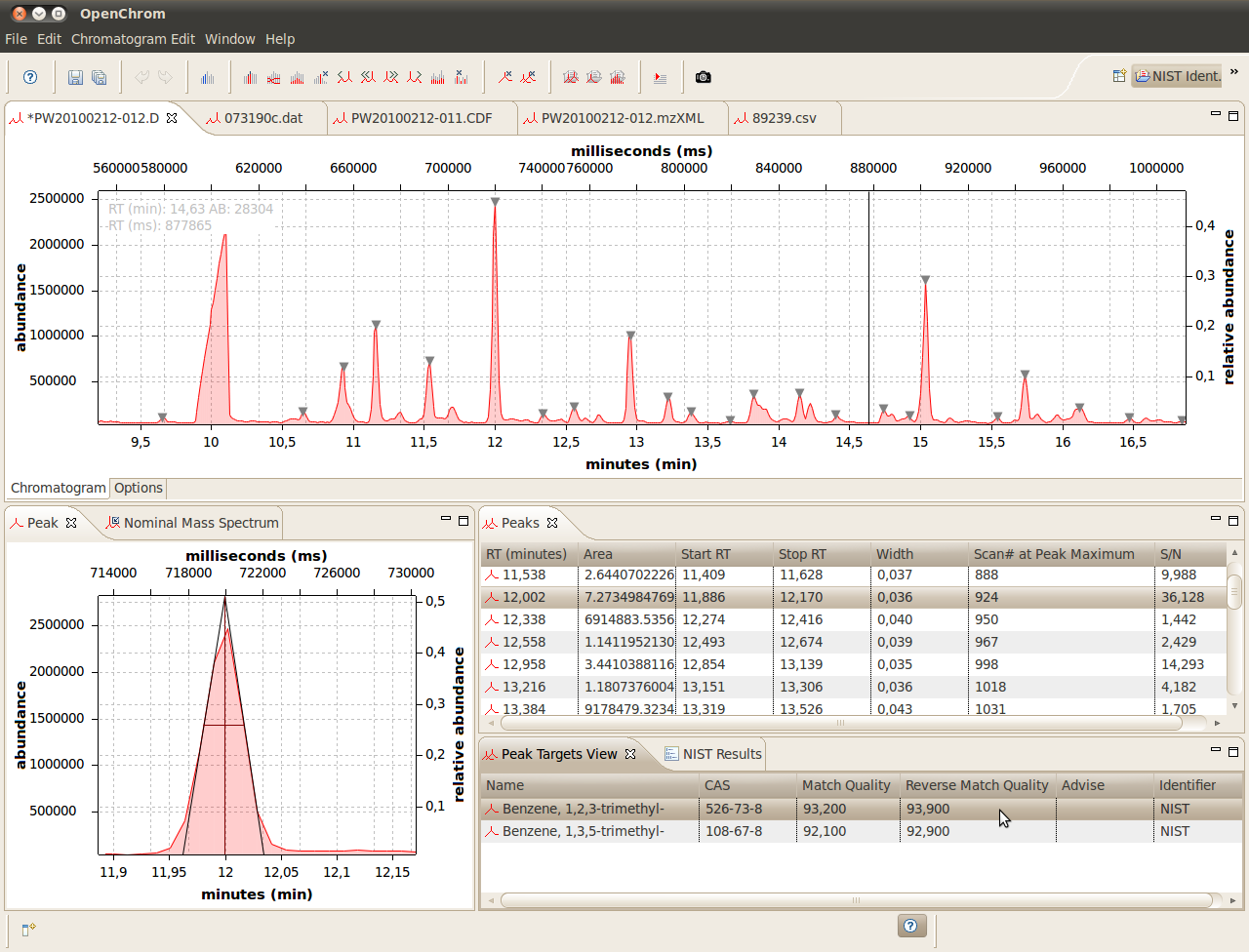For gas chromatography and mass spectrometry specifically, Philip Wenig's OpenChrom is an open source data system that can import binary and textual chromatographic data files directly. It includes methods to detect baselines and to measure peak areas in a chromatogram. Extensive documentation is available. It is available for Windows, Linux, Solaris and Mac OS X.  A screen shot is shown on the left (click to enlarge). The program and its documentation is regularly updated by the author.

Another freely-available open-source program for mass spectroscopy is "Skyline" from MacCoss Lab Software, which is specifically aimed at reaction monitoring. Tutorials and videos are available.

Peak area measurement using spreadsheets.EffectOfDx.xlsx (screen image) demonstrates that the simple equation sum(y)*dx accurately measures the peak area of an isolated Gaussian peak if there are at least 4 or 5 points visibly above the baseline (or 2.5 points within the 4*sigma basewidth) and as long as you include the points out to plus and minus at least 2 or 3 standard deviations of the Gaussian. It also shows that an exponentially broadened Gaussian needs to include more points on the tailing (right-hand, in this case) side to achieve the best accuracy.  EffectOfNoiseAndBaseline.xlsx (screen image) demonstrates the effect of random noise and non-zero baseline, showing that the area is more sensitive to non-zero baseline that the same amount of random noise.

CumulativeSum.xls (screen image) illustrates integration of a peak-type signal by normalized cumulative sum; you can paste your own data into columns A and B. CumulativeSumExample.xls is an example with data . The Excel and Calc spreadsheets PeakDetectionAndMeasurement and CurveFitter can measure the areas under partly overlapping Gaussian peaks in time-series data, using the findpeaks algorithm and iterative non-linear curve fitting techniques, respectively. But neither is as versatile as using a dedicated chromatography program such as OpenChrom.

Using sharpening for overlapping peak area measurements. There is a set of downloadable spreadsheets for perpendicular drop area measurements of overlapping peaks using 2nd and 4th derivative sharpening. Sharpening the peaks reduces the degree of overlap and can greatly reduce the peak area measurement error errors made by the perpendicular drop method. There is an empty template for you to paste your data into (PeakSharpeningAreaMeasurementTemplate.xlsm), an example version with sample data and settings already entered (PeakSharpeningAreaMeasurementExample.xlsm), and a "demo" that creates and measures simulated data with known areas (PeakSharpeningAreaMeasurementDemo.xlsm) so you can see how sharpening effects area measurement accuracy. There are very brief instructions in row 2 of each of these. Also, there are mouse-over pop-up notes on many of the cells (indicated by a red marker in the upper right corner of the cell). All three have clickable ActiveX buttons for convenient interactive adjustment of the K2 and K4 factors by 1% or by 10% for each click. Of course the problem is knowing what values of the 2nd and 4th derivative weighting factors (K1 and K2) to use. Those values depend on the peak separation, peak width, and the relative peak height of the two peaks, and they must be selected experimentally based on your preferred trade-off between extent of sharpening and extent of baseline upset. A good place to start for Gaussian peaks is (sigma^2)/30 for the 2nd derivative factor and (sigma^4)/200 for the 4th derivative factor, where sigma is the standard deviation of the Gaussian, then adjust to give the narrowest peaks without significant negative dips. Don't assume that increasing the K's until baseline resolution is achieved will always give the best area accuracy. The optimum values depend on the ratio of peak heights: at 1:1, with equal widths and shapes, the perpendicular drop method works perfectly with no sharpening, but if there is inequality in shapes, heights, or widths, increased K values give lower errors up to a point, but overdoing the sharpening can sacrifice accuracy. The two screen images screen1 and screen2, which use the same K values, show that it is possible to find K values that give excellent accuracy for peak 2 over a range of relative peak heights, even when the smaller peak is quite small. Without sharpening, accurate perpendicular drop area measurements are impossible because there is no valley between the peaks.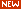The symmetrization of exponentially broadened peaks by the weighted addition of the first derivative is performed by the template PeakSymmetrizationTemplate.xlsm (graphic); PeakSymmetrizationExample.xlsm is an example application with sample data already typed in. The procedure here is first to adjust k1 to get the most symmetrical peak shapes (judged by equal but opposite slopes on the leading and trailing edges), then enter the start time, valley time, and end time from the graph for the pair of peaks you want to measure into cells B4, B5, and B6, and finally (optionally) adjust the second derivative sharpening factor k2. The perpendicular drop areas of those two peaks are reported in the table in columns F and G. These spreadsheets have Active-X clickable buttons to adjust the first derivative weighting factor (k1) in cell J4 and the second derivative sharpening factor k2 (cell J5). There is also a demo version that allows you to determine the accuracy of perpendicular drop peak areas under different conditions by internally generating overlapping peaks of known peak areas, with specified asymmetry (B6), relative peak height (B3), width (B4), and noise (B5): PeakSymmetrizationDemo.xlsm (graphic). For peaks that have a more complex broadening behavior, the template PeakDoubleSymmetrizationExample.xlsm allows symmetrization of double exponential broadening (graphic).

Peak area measurement using Matlab and Octave. and Octave have built-in commands for the sum of elements ("sum", and the cumulative sum "cumsum") and the trapezoidal numerical integration ("trapz").  For example, these three Matlab commands

>> x=-5:.1:5;
>> y=exp(-(x).^2);
>> trapz(x,y)

These lines accurately compute the area under the curve of x,y (in this case an isolated Gaussian, whose area is theoretically known to be the square root of pi, sqrt(pi), which is 1.7725. If the interval between x values, dx, is constant, then the area is simply yi=sum(y).*dxAlternatively, the signal can be integrated using yi=cumsum(y).*dx, then the area of the peak will be equal to the height of the resulting stepmax(yi)-min(yi)=1.7725.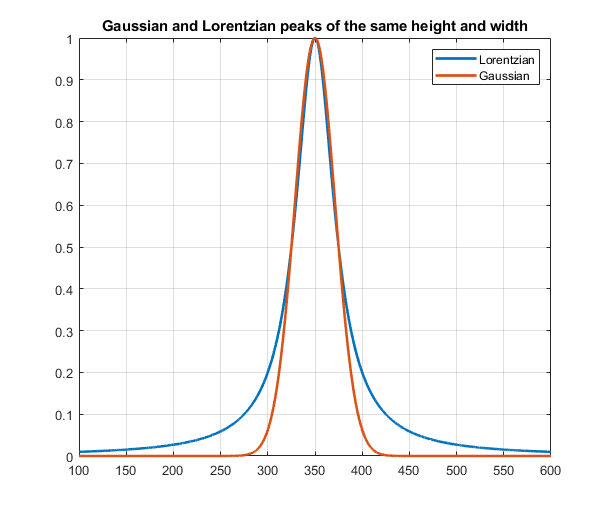The area of a peak is proportional to the product of its height and its width, but the proportionality constant depends on the peak shape. A pure Gaussian peak with a peak height h and full-width at half-maximum w has a total area of 1.064467*h*w. A Gaussian-Lorentzian blend with p percent Gaussian character has an area of ((100- p)/100)*((pi/2).*w*h) + (p /100)*(1.064467*w*h). A pure Lorentzian peak has a total area of (pi/2)*h*w. The graphic LorentzianVsGaussian.png compares Gaussian and Lorentzian peaks of the same height and width. The Lorentzian has more area in the outer wings, so if you measure the area of an unknown peak using trapz, you have to measure over a very wide range on both sides of the peak. To get an area within 1%, you need to expand that to 64 times the FWHM! (See LorentzianAreaProblem.m, graphic). Many real signals in practice have too many peaks that are too close together to allow the theoretical areas to be measured directly by integration. If you really need an accurate area and the available measurement span is insufficient, it may be more accurate to calculate the area analytically using the above analytical expressions.

For a peak of any smooth shape on a zero baseline, the peak width (FWHM) can be measured by my halfwidth.m function.

But the peaks in real signals have some complications:
(a) their shapes might not be known;
(b) they may be superimposed on a baseline; and
(c) they may be overlapped with other peaks.
These must be taken into account to measure accurate areas in experimental signals. Various Matlab/Octave functions have been developed to deal with these complications.

Overlapping peaks. The following Matlab/Octave code uses the perpendicular drop (PD) method to measure the areas of two overlapping symmetrical peaks in the data vectors x,y by the perpendicular drop method. Variables "m1" and "m2" are the estimated positions of the two peaks. The "val2ind" function returns the index number of the value in a vector that value matches the specified value.

The third line finds the half-way point between the two peaks. The last two lines use the trapz function to measure the areas before and after the valley point.

index1=val2ind(x,m1);
index2=val2ind(x,m2);
valleyindex=val2ind(x,(m1+m2)/2)
,
PDMeasArea1=trapz(x(1:valleyindex),y(1:valleyindex));
PDMeasArea2=trapz(x(valleyindex:length(x)),y(valleyindex:length(x));

Alternatively, you could replace "valleyindex" with valleyy=min(y(index1:index2)); valleyindex=val2ind(y,valleyy); which uses the minimum between the peaks rather than the half-way point. But the half-way point method has the advantage that the SNR at a signal maximum is usually better than at a minimum, so it's likely that maxima are more precisely located that minima. Moreover, the half-way point method works even when the overlap is so great that there is not a discernible minimum between the peaks. My function PerpDropAreas.m uses the half-way point method to measure the areas of any number of overlapping peaks, given a list of their peak maxima positions. These methods work best if the peak widths are not very different.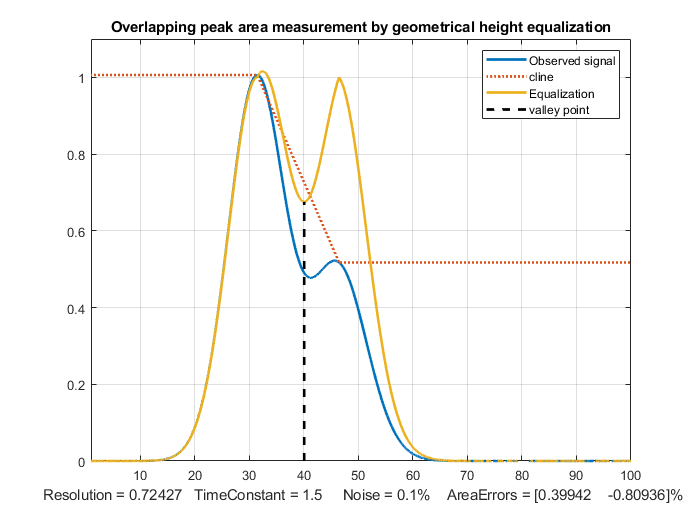Although the perpendicular drop method remains popular, there are other geometrical methods that can work better in many cases. The "equalization" method, illustrated in the figure on the left, uses another method of locating the perpendicular drop point. A line of three straight-line segments
is constructed that touches the estimated maxima of the two peaks, shown by the dotted red line called the "cline" in the figure on the left. The quotient of the original signal, in blue, divided by this line, results in a temporarily normalized signal (the yellow line) that has more nearly equal peak heights. The effect of this treatment is to deepen the valley between the peaks, so that it remains distinct for lower values of the second peak height. This is used only for the purpose of determining its minimum, shown as a vertical black line, and then is discarded. Using that minimum as the separation point between the peaks, the perpendicular drop areas are then calculated on the original observed signal (blue line). Note that this new valley point is not quite the same as the valley of the original signal, nor is it the half-way point between the two peak positions. The process need not be done by hand; it is easily automated, given only an initial estimate for the two peak positions based on the observed signal. The Matlab/Octave function EqualPerpDrop.m performs the calculation; the script EqualPerpDropTest.m demonstrates the use of the function applied to the measurement of two simulated overlapping EMG (exponentially modified Gaussian) peaks.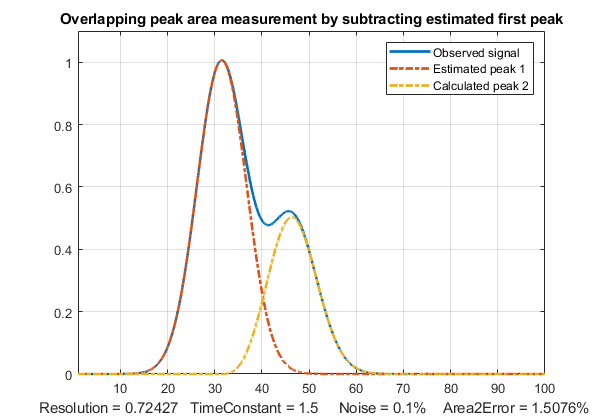The "reflection/subtraction" method, shown on the right, is simpler. As before, the original signal is shown in blue. An estimate o
f the isolated first (larger) peak is constructed by reflecting its left half and using it to replace the right half, resulting in the red dotted line in the figure, assuming that the first peak is symmetrical. Then that peak is simply subtracted from the entire signal to reveal the isolated second peak (dotted yellow line). The two areas are then separately calculated by the "trapz" function. This process is also easily automated, given only the peak position of the first peak. It works perfectly only if the first peak is the larger of the two peak and is symmetrical and if the peak separation is sufficient so that the left-hand tail of the smaller peak does not significantly increase the height of the first peak.

So, how do these new methods compare to the traditional perpendicular drop method? Matlab/Octave code for all of these methods is contained in the script "OverlapAreaComparison.m". For the case of Gaussian peak with a resolution of 0.7 and a height ratio of 1 to 0.5, the relative percent error of the peak areas are:
```                                    Peak 1 Peak 2
Perpendicular drop, valley point:  -6.44%  12.89%Perpendicular drop, half-way point: 3.91%  -7.83%Equalization method:                1.27%  -2.54%Subtraction method:                -2.12%   4.25%```

You can change the parameters in lines 5 through 10 to test with other peak separations and relative peak heights. The equalization method is often, but not always, the most accurate method. (Note: the script requires the downloadable functions val2ind.m, halfwidth.m, ExpBroaden.m, and plotit.m functions be in the path).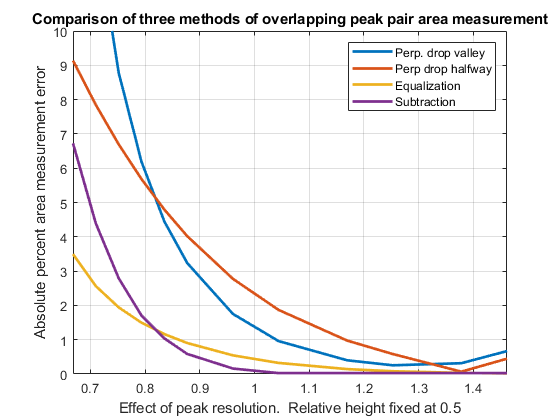A more through investigation of these methods demonstrates the effect of changing the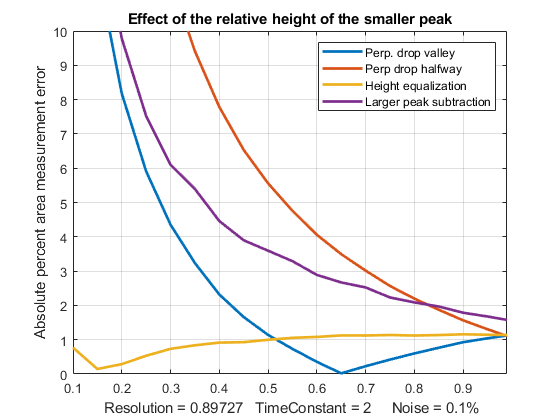peak resolution, shown on the left (script, graphic) and of changing the the height of the smaller peak, shown on the right (script, graphic). These scripts include the effect of random noise in the signal, because noise can influence the location of peak maxima and the separation point between the peaks, whether they are determined manually or by a computer algorithm (as it is here)
; the random noise is set by the variable "noise", which is the fractional random white noise added to the signal. Also, these scripts include the effect of asymmetry of the peak shapes, which can cause errors in area measurement by all these methods. After all, the very reason for measuring peak area rather than peak heights is to reduce the effect of uncontrolled variations in peak broadening The asymmetry is set by the variable "TimeConstant", which is the time constant of the exponential convolution applied to the signal that reduces the height and stretches out the right-hand half. Both of those are zero in the above figures for simplicity and to show the best possible accuracy. For example, with a resolution of 1.0, a tau of 2, and noise set to 0.01 (1%), the valley perpendicular drop and the equalization method outperform the other methods (graphic).

Things are much easier and more forgiving in quantitative analysis, using a calibration curve, because
in that case absolute area accuracy is not really necessary. Rather, it is really the reproducibility of the areas that is key. Systematic errors in the area measurement simply change the slope of the calibration curve, and as long as the conditions are the same between calibration and analysis (always a requirement in any case), the error will cancel out exactly. For example, if you run the above scripts with very asymmetrical peaks (TimeConstant=3), poor resolution (resolution =0.68), and visible amounts of random noise=5%, the systematic area measurement errors are quite large (5%-15%), but nevertheless good linear calibration curves are produced by both the halfway point perpendicular drop and the equalization method, over the range of relative peak heights of 0.1 to 0.99, with correlation coefficients of 0.999.

All of these methods produce significant errors if the peaks are highly overlapped or asymmetrical. However, asymmetry that is the
result of exponential broadening can be symmetrized before computing the areas using the first derivative addition method, which sharpens the peaks and removes the asymmetry without changing the peak areas. Other methods of peak sharpening, especially self-deconvolution, can also be used when the peak to be measured is too weak or too poorly resolved to allow easy measurement. Ultimately, in the most difficult cases, you may have to consider the use of iterative curve fitting, though it is admittedly more complex mathematically and is subject to its own limitations.

Automatic multiple peak detection

Measurepeaks.m is a function that quickly and automatically detects peaks in a s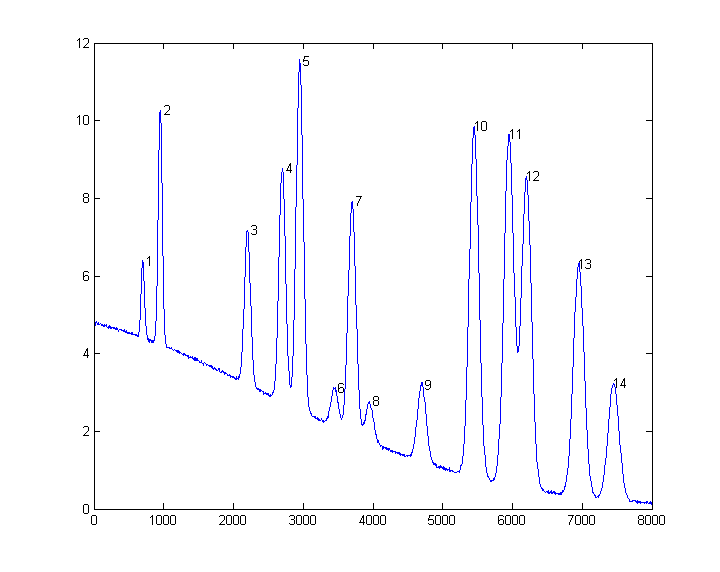ignal, using the derivative zero-crossing method described previously, and measures their areas using the perpendicular drop and tangent skim methods. It shares the first 6 input arguments with findpeaksSG. The syntax is M=measurepeaks(x,y, SlopeThreshold, AmpThreshold, SmoothWidth, FitWidth, plots). It returns a table containing the peak number, peak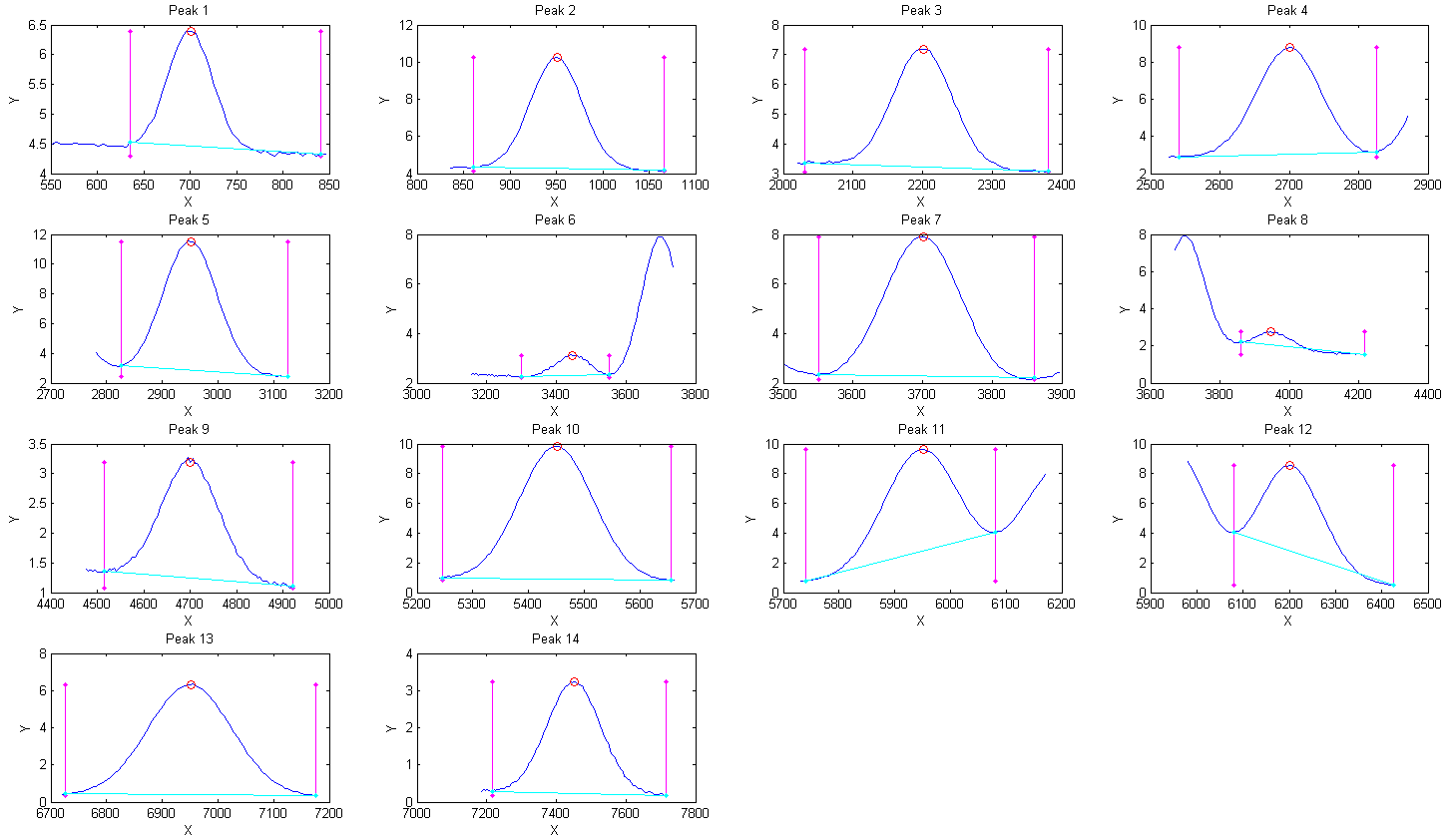position, absolute peak height, peak-valley difference, perpendicular drop area, and the tangent skim area of each peak it detects. If the last input argument ('plots') is set to 1, it plots the signal with numbered peaks (shown on the left) and also plots the individual peaks (in blue) with the maximum (red circles), valley points (magenta), and tangent lines (cyan) marked as shown on the right. Type "help measurepeaks" and try the seven examples there, or run HeightAndArea.m to test the accuracy of peak height and area measurement with signals that have multiple peaks with noise, background, and some peak overlap. Generally, the values for absolute peak height and perpendicular drop area are best for peaks that have no background, even if they are slightly overlapped, whereas the values for peak-valley difference and for tangential skim area are better for isolated peaks on a straight or slightly curved background. Note: this function uses smoothing (specified by the SmoothWidth input argument) only for peak detection; it performs measurements on the raw unsmoothed y data. If the raw data are noisy, it may be beneficial to smooth the y data yourself before calling measurepeaks.m, using any smooth function of your choice.

[M,A]=autopeaks.m is basically a combination or autofindpeaks.m and measurepeaks.m. It has similar syntax to measurepeaks.m, except that the peak detection parameters (SlopeThreshold, AmpThreshold, smoothwidth  peakgroup, and smoothtype) can be omitted and the function will calculate trial values in the manner of autofindpeaks.m. Using the simple syntax
[M,A]=autopeaks(x, y) works well in some cases, but if not try [M,A]=autopeaks(x, y, n), using different values of n (roughly the number of  peaks that would fit into the signal record) until it detects the peaks that you want to measure. Like measurepeaks, it returns a table M containing the peak number, peak position, absolute peak height, peak-valley difference, perpendicular drop area, and tangent skim area of each peak it detects, but is also can optionally return a vector A containing the peak detection parameters that it calculates (for use by other peak detection and fitting functions). For the most precise control over peak detection, you can specify all the peak detection parameters by typing M=autopeaks(x,y, SlopeThreshold, AmpThreshold, smoothwidth, peakgroup). [M,A]=autopeaksplot.m is the same but it also plots the signal and the individual peaks in the manner of measurepeaks.m (shown above).

The script testautopeaks.m runs all the examples in the autopeaks help file, with a 1-second pause between each one, printing out results in the command window and additionally plotting and numbering the peaks (Figure window 1) and each individual peak (Figure window 2); it requires gaussian.m and fastsmooth.m in the path.

Peak sharpening, introduced previously, can often help in the measurement of the areas of overlapping peaks, by creating (or deepening) the valleys between peaks that are needed by the perpendicular drop method.SharpenedOverlapDemo.m is a script that automatically determines the optimum degree of even-derivative sharpening that minimizesthe errors of measuring peak areas of by the perpendicular drop method using the autopeaks.m function. It does this by applying different degrees of sharpening and plotting the area errors (percent difference between the true and measured errors) vs the sharpening factor, as shown on the right. It also shows the height of the valley between the peaks (yellow line). This demonstrates that (1) the optimum sharpening factor depends upon the width and separation of the two peaks and on their height ratio, (2) that the degree of sharpening is not overly critical, often exhibiting a broad optimum region, (3) that the optimum for the two peaks is not necessarily exactly the same, and (4) that the optimum for area measurement usually does not occur at the point where the valley is zero. (To run this script you must have gaussian.m, derivxy.m, autopeaks.m, val2ind.m, and halfwidth.m in the path. Download these from https://terpconnect.umd.edu/~toh/spectrum/).

SharpenedOverlapCalibrationCurve.m is a script that simulates the construction and use of calibration curves of three overlapping Gaussian peaks (the blue lines in the signal plots on the left) . Even-derivative sharpening (the red line in the signal plots) is used to improve the resolution of the peaks to allow perpendicular drop area measurement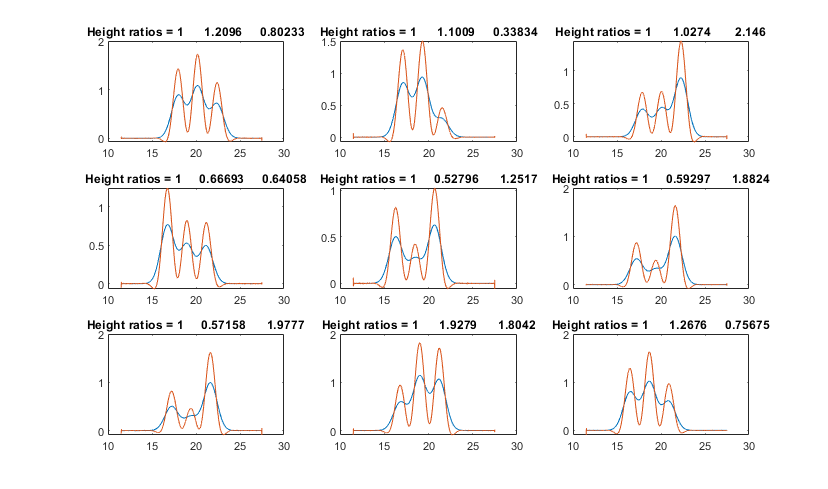. A straight line is fit to the calibration curve and the R2 is calculated, in order to demonstrate (1) the linearity of the response, and (2) in independence of the overlapping  adjacent peaks. You can easily change:

1. The resolution, Rs, by changing the peak width w in line 15. Default is w=2, Rs=0.55
2. The peak ratios, by changing the minimum and maximum peaks in lines 21 and 22. Default is 0.2 and 1.0. (1:5 ratio range)
3. The number of standards in line 24. Larger numbers give a more reliable calibration curve.
4. The number of simulated samples, line 25. Larger numbers give more reliable average errors.

SymmetrizedOverlapCalibrationCurve.m is the same thing for symmetrization of overlapping exponentially modified Gaussian peaks by first-derivative addition. The critical variable is "factor" in line 27, which for best results should match or slightly exceed "tau", the exponential time constant in line 19. To compare to using the original signal, set "factor" to 0.1. You must have gaussian.m, derivxy.m, autopeaks.m, val2ind.m, halfwidth.m, fastsmooth.m, and plotit.m in the path for either of these two scripts.

The Matlab/Octave automatic peak-finding function findpeaksG.m computes peak area assuming that the peak peak shape is Gaussian (or Lorentzian, for the variant findpeaksL.m). The related function findpeaksT.m uses the triangle construction method to compute the peak parameters. Even for well-separated Gaussian peaks, the area measurements by the triangle construction method is not very accurate; the results are about 3% below the correct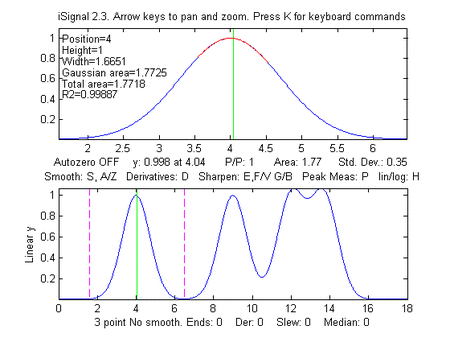values. (But this method does perform better than findpeaksG.m when the peaks are noticeably asymmetric; see triangulationdemo for some examples). In contrast, measurepeaks.m makes no assumptions about the shape of the peak.; it simply looks for the minima on both sides of each peak to use as the dividing point between peaks.

iSignal (shown on the left) is a downloadable interactive multipurpose signal processing Matlab function that includes various signal processing functions described in this tutorial, including measurement of peak area using Simpson's Rule and the perpendicular drop method. Click to view or right-click > Save link as...  here, or you can download the ZIP file with sample data for testing.
It is shown on the left applying the perpendicular drop method to a series of four peaks of equal area. (Look at the bottom panel to see how the measurement intervals, marked by the vertical dotted magenta lines, are positioned at the valley minimum on either side of each of the four peaks).

Here's a bit of Matlab/Octave code that creates
four computer-synthesized Gaussian peaks, similar to this figure, that all have the same height (1.000), width (1.665), and area (1.772) but with different degrees of peak overlap:

x=[0:.01:18];
y=exp(-(x-4).^2) + exp(-(x-9).^2) + exp(-(x-12).^2) + exp(-(x-13.7).^2);
isignal(x,y);

To use iSignal to measure the areas of each of these peaks by the perpendicular drop method, use the pan and zoom keys to position the two outer cursor lines (dotted magenta lines) in the valley on either side of the peak. The total of each peak area will be displayed below the upper window.

Peak #   Position       Height     Width      Area
1       4.00         1.00       1.661      1.7725
2       9.001        1.0003     1.6673     1.77
3      12.16         1.068      2.3        1.78
4      13.55         1.0685     2.21       1.79

The area results are reasonably accurate in this example only because the perpendicular drop method roughly compensates for partial overlap between peaks, but only if the peaks are symmetrical, about equal in height, and have zero background.

iSignal version 5.9 includes an additional command (J key) that calls the autopeaksplot function, which automatically detects the peaks in the signal and measures their peak position, absolute peak height, peak-valley difference, perpendicular drop area, and tangent skim area. It asks you to type in the peak density (roughly the number of peaks that would fit into the signal record); the greater this number, the more sensitive it is to narrow peaks. It displays the measured peaks just as does the measurepeaks function described above. (To return to iSignal, press any cursor arrow key).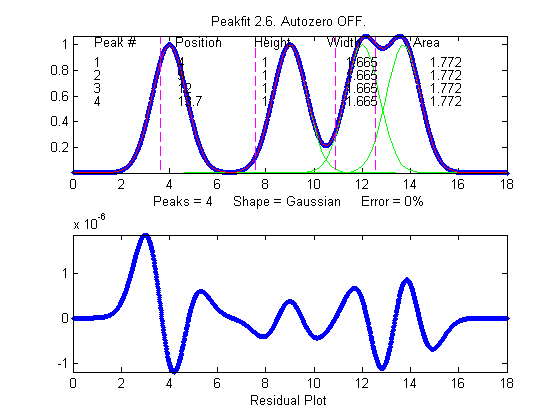Area measurement by iterative curve fitting. In general, the most flexible peak area measurements for overlapping peaks, assuming that the basic shape of the peaks is known or can be guessed, are made with iterative least-squares peak fitting, for example using peakfit.m, shown on the right (for Matlab and Octave). This function can fit any number of overlapping peaks with model shapes selected from a list of different types. It uses the "trapz" function to calculate the area of each of the component mode peak.

For example, using the peakfit function on the same data set as above, the results are much more accurate:

>> peakfit([x;y],9,18,4,1,0,10,0,0,0)

Peak #    Position      Height       Width        Area
1            4            1       1.6651       1.7725
2            9            1       1.6651       1.7725
3           12            1       1.6651       1.7725
4         13.7            1       1.6651       1.7725

iPeak can also be used to estimate peak areas. It uses the same Gaussian curve fitting method as iSignal, but it has the advantage that it can detect and measure all the peaks in a signal in one operation.  For example:

>> ipeak([x,y],10)

Peak #    Position      Height       Width        Area
1            4            1       1.6651       1.7727
2       9.0005       1.0001       1.6674       1.7754
3        12.16       1.0684       2.2546       2.5644
4        13.54       1.0684       2.2521       2.5615

Peaks 1 and 2 are measured accurately by iPeak, but the peak widths and areas for peaks 3 and 4 are not accurate because of the peak overlap.  Fortunately, iPeak has a built-in "peakfit" function (activated by the N key) that uses these peak position and width estimates as its first guesses, resulting in good accuracy for all four peaks.

Fitting Error 0.0002165%
Peak#     Position     Height      Width         Area
1            4            1       1.6651       1.7724
2            9            1       1.6651       1.7725
3           12            1       1.6651       1.7725
4         13.7      0.99999       1.6651       1.7724Correction for background/baseline. The presence of a baseline or background signal, on which the peaks are superimposed, will greatly influence the measured peak area if not corrected or compensated. iSignal, iPeak, measurepeaks, and peakfit all have several different baseline correction modes, for flat, linear, and quadratic baselines, and iSignal and iPeak have a multipoint piece-wise linear baseline subtraction function allows the manually estimated background to be subtracted from the entire signal.  See iSignal.html#background_subtraction, ipeakdemo1 on PeakFindingandMeasurement.htm#demos, and CurveFittingC.html#Background_correction for examples of these background correction functions. If the baseline is actually caused by the edges of a strong overlapping adjacent peak, then it's possible to include that peak in the curve-fitting operation, as see in Example 22 on InteractivePeakFitter.htm.

The script AreasOfIsolatedPeaks2.m demonstrates the use of peakfit.m for a simulated experimental signal consisting of several isolated peaks on a straight tilted baseline. In this example, the positions of the peaks are assumed to have been previously measured by a peak detector algorithm and to be reproducible enough that a pre-determined set of measurement segments can be used to fit each peak separately and determine its position, height, width, and area.

Here's a Matlab/Octave experiment that compares several different methods of baseline correction in peak area measurement. The signal consists of two noiseless, slightly overlapping Gaussian peaks with theoretical peak heights of 2.00 and 1.00 and areas of 191.63 and 95.81 units, respectively. The baseline is tilted and linear, and slightly greater in magnitude than the peak heights themselves, but the most serious problem is that the signal never returns to the baseline long enough to make it easy to distinguish the signal from the baseline.

>> x=400:1:800;y=2.*gaussian(x,500,90)+1.*gaussian(x,700,90)+2.*(x./400);

iSignal, using perpendicular drop in baseline mode 1, seriously underestimates both peak areas (168.6 and 81.78).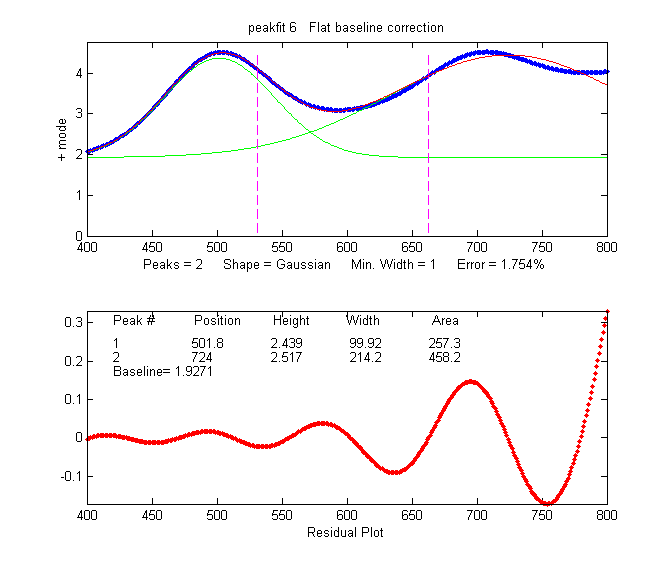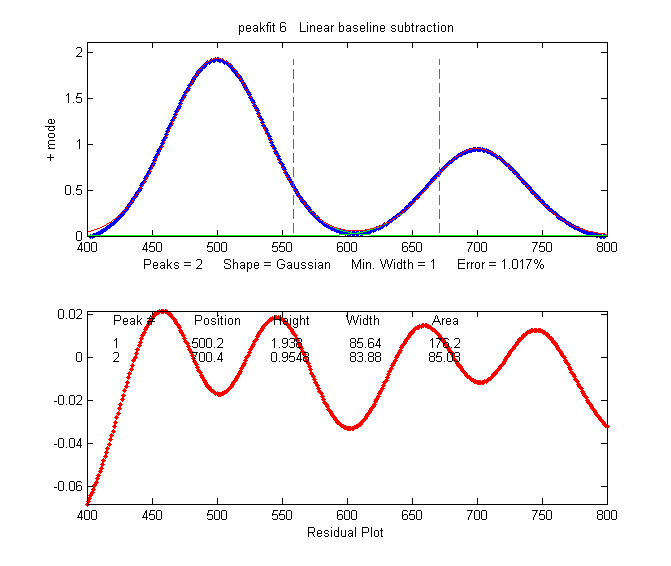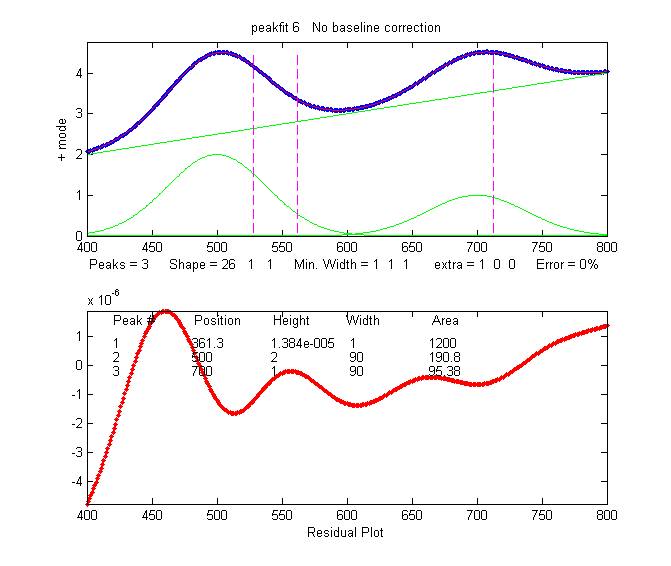An automated tangent skim measurement by measurepeaks is not accurate in this case because the peaks do not go all the way down to the baseline at the edges of the signal and because of the slight overlap:

>> measurepeaks(x,y,.0001,.8,2,5,1)
Position  PeakMax  Peak-valley  Perp drop  Tan skim
1   503.67    4.5091     1.895       672.29     171.44
2   707.44    4.5184    0.8857       761.65     76.685

An attempt to use curve fitting with peakfit.m in the flat baseline correction mode 3 - peakfit([x;y],0,0,2,1,0,1,0,3), above, left-most figure - does not really work because the actual baseline is tilted, not flat. The linear baseline mode does a little better (peakfit([x;y],0,0,2,1,0,1,0,1), second figure from left) but it's not perfect in this case. A more accurate approach is to fit the baseline as a third "peak" of a different shape, with either a Lorentzian model - peakfit([x;y],0,0,3,[1 1 2]), third signal from the left - or with a "slope" model - shape 26 in peakfit version 6, last figure on the right. The latter method gives both the lowest fitting error (less than 0.01%) and the most accurate peak areas (less than 1/2% error in peak area):

>> [FitResults,FitError]=peakfit([x;y],0,0,3,[1 1 26])
FitResults =
1          500       2.0001       90.005       190.77
2          700      0.99999       89.998       95.373
3       5740.2  8.7115e-007            1       1200.1

FitError =0.0085798

Note that in this last case the number of peaks is 3 and the shape argument is a vector [1 1 26] specifying two Gaussian components plus the "linear slope" shape 26. If the baseline seems to be non-linear, you might prefer to model it using a quadratic (shape 46; see example 38 on InteractivePeakFitter.htm#Examples). If the baseline seems to be different on either side of the peak, try modeling the baseline with an S-shape (sigmoid), either an up-sigmoid, shape 10 (click for graphic), peakfit([x;y],0,0,2,[1 10],[0 0]), or a down-sigmoid, shape 23 (click for graphic), peakfit([x;y],0,0,2,[1 23],[0 0]), in these examples leaving the peak modeled as a Gaussian.Asymmetrical peaks and peak broadening: perpendicular drop vs curve fitting.

AsymmetricalAreaTest.m is a Matlab/Octave script that compares the accuracy of peak area measurement methods for a single noisy asymmetrical peak measured by different methods: (A) Gaussian estimation,(B) triangulation, (C) perpendicular drop method, and curve fitting by (D) exponentially broadened Gaussian, and (E) two overlapping Gaussians. AsymmetricalAreaTest2.m is similar except that it compares the precision (standard deviation) of the areas. For a single peak with zero baseline, the perpendicular drop and curve fitting methods work equally well, both considerably better than Gaussian estimation or triangulation. The advantage of the curve fitting methods is that they can deal more accurately with peaks that overlap or that are superimposed on a baseline.

Here's a Matlab/Octave experiment that simulates a signal containing five Gaussian peaks with the same initial peak height (1.0) and width (3.0) but which are subsequently broadened by increasing degrees of exponential broadening, similar to the broadening of peaks commonly encountered in chromatography:>> x=5:.1:65;
>> y=modelpeaks2(x, [1 5 5 5 5], [1 1 1 1 1], [10 20 30 40 50], [3 3 3 3 3],
[0 -5 -10 -15 -20]);
>> plot(x,y)

The theoretical area under these Gaussians is all the same: 1.0645*Height*Width = 1*3*1.0645= 3.1938. A perfect area-measuring algorithm would return this number for all five peaks.

As the broadening is increased from left to right, the peak height decreases (by about 35%) and peak width increases (by about 32%). But because the area under the peak is proportional to the product of the peak height and the peak width, these two changes approximately cancel each other out and the result is that the peak area is nearly independent of peak broadening (see the summary of results in 5ExponentialBroadenedGaussianFit.xlsx). The Matlab/Octave peak-finding function findpeaksG.m, finds all five peaks and measures their areas assuming a Gaussian shape; this works well for the unbroadened peak 1 (script), but it underestimates the areas as the broadening increases in peaks 2-5:

Peak   Position    Height     Width     Area
1       10.0000    1.0000    3.0000    3.1938
2
20.4112    0.9393    3.1819    3.1819
3
30.7471    0.8359    3.4910    3.1066
4
40.9924    0.7426    3.7786    2.9872
5
51.1759    0.6657    4.0791    2.8910

The triangle construction method (using
findpeaksT.m) underestimates even the area of the unbroadened peak 1 and is less accurate for the broadened peaks (script; graphic):

Peak   Position    Height     Width     Area
1       10.0000    1.1615    2.6607    3.0905
2
20.3889    1.0958    2.8108    3.0802
3
30.6655    0.9676    3.1223    3.0210
4
40.8463    0.8530    3.4438    2.9376
5
50.9784    0.7563    3.8072    2.8795

The automated function measurepeaks.m gives better results using the perpendicular drop method (5th column of table).

M=measurepeaks(x,y,0.0011074,0.10041,3,3,1)
`  Peak Position PeakMax  Peak-val. Perp drop Tan skim  1    10        1       .99047    3.1871    3.1123  2    20.4      .94018  .92897    3.1839    3.0905    3    30.709    .83756  .81805    3.1597    2.9794  4    40.93     .74379  .70762    3.1188    2.7634  5    51.095    .66748  .61043    3.0835    2.5151`
Using iSignal and the manual peak-by-peak perpendicular drop method yields areas of 3.193, 3.194, 3.187, 3.178, and 3.231, a mean of  3.1966 (pretty close to the theoretical value of 3.1938) and standard deviation of only 0.02 (0.63%). Alternatively, integrating the signal, cumsum(y).*dx), where dx is the difference between adjacent x-axis values (0.1 in this case), and then measuring the heights of the resulting steps, gives similar results: 3.19, 3.19, 3.18, 3.17, 3.23. By either method, the peak heights are very different but the areas are closer together, yet not exactly equal.

But we can obtain a more accurate automated measurement of all five peaks by iterative curve fitting, using peakfit.m with multiple shapes, one Gaussian and four exponentially modified Gaussians (shape 5) with different exponential factors (extra vector):

>> [FitResults,FittingError]=peakfit([x;y],30,54,5,[1 5 5 5 5],[0  -5 -10 -15 -20],10, 0, 0)
FitResults =
Peak#   Position     Height       Width         Area
1        9.9933     0.98051       3.1181       3.2541
2       20.002      1.0316        2.8348       3.1128
3       29.985      0.95265       3.233        3.2784
4       40.022      0.9495        3.2186       3.2531
5       49.979      0.83202       3.8244       3.2974
FittingError =
2.184%
The results in this case are disappointing; the fitting error is not much better than the simple Gaussian fit.

Better results can be had using preliminary position and width results obtained from the findpeaks function or by curve fitting with a simple Gaussian fit and using those results as the "start" vector:

>> [FitResults,FittingError]=peakfit([x;y],30,54,5, [1 5 5 5 5], [0  -5 -10 -15 -20], 10, [10 3.5 20 3.5 31 3.5 41 3.5 51 3.5], 0)
FitResults =
Peak#   Position       Height       Width        Area

1        9.9999     0.99995      3.0005       3.1936

2       20          0.99998      3.001        3.1944

3       30.001      1.0002       3.0006       3.1948

4       40          0.99982      2.9996       3.1924

5       49.999      1.0001       3.003        3.1243
FittingError =
0.02%

Even more accurate results for area are obtained using peakfit with one Gaussian and four equal-width exponentially modified Gaussians (shape 8):

>> [FitResults,FittingError]=peakfit([x;y],30,54,5, [1 8 8 8 8], [0  -5 -10 -15 -20],10, [10 3.5 20 3.5 31 3.5 41 3.5 51 3.5],0)
FitResults =
Peak#  Position    Height        Width          Area
1    10        1.0001         2.9995        3.1929
2    20        0.99998        3.0005        3.1939

3    30        0.99987        3.0008        3.1939

4    40        0.99987        2.9997        3.1926
5    50        1.0006         2.9978        3.1207
FittingError =
0.008%
The latter approach works
because, although the broadened peaks clearly have different widths (as shown in the simple Gaussian fit), the underlying pre-broadening peaks have all the same width. In general, if you expect that the peaks should have equal widths, or fixed widths, then it's better to use a constrained model that fits that knowledge; you'll get better estimates of the measured unknown properties, even though the fitting error will be higher than for an unconstrained model.

The disadvantages of the exponentially-broadened model are that (a) it may not be a perfect match to the actual physical broadening process; (b) it's slower that a simple  Gaussian fit, and (c) it sometimes need help, in the form of a start vector or equal-widths constraints, as seen above, in order to get the best results.

Alternatively, if the objective is only to measure the peak areas, and not the peak positions and widths, then it's not even necessary to model the physical peak-broadening of each peak. You can simply aim for a good fit using two (or more) closely-spaced simple Gaussians for each peak and simply add up the areas of the best-fit model. For example, the 5th peak in the above example (the most asymmetrical), can be fit very well with two overlapping Gaussians, resulting in a total area of  1.9983+1.1948 = 3.1931, very close to the theoretical area of 3.1938.  Even more overlapping Gaussians can be used if the peak shape is more complex. This is called the "sum rule" in integral calculus: the integral of a sum of two functions is equal to the sum of their integrals. As a demonstration, the script SumOfAreas.m is shows that even drastically non-Gaussian peaks can be fit with multiple Gaussian components, and that the total area of the components approaches the area under the non-Gaussian peak as the number of components increases (graphic). When using this technique, it's best to set the number of trials (NumTrials, the 7th input argument of the peakfit.m function) to 10 or more; additionally, if the peak of interest is on a baseline, you must add up the areas of only those peak that contribute to fitting the peak itself and not those that are fitting the baseline.

By stimulating a case where the peaks are closer together, we can create a tougher and more realistic challenge.y=modelpeaks2(x,[1 5 5 5 5],[1 1 1 1 1],[20 25 30 35 40],[3 3 3 3 3],[0 -5 -10 -15 -20]);

In this case, the triangle construction method gives areas of [3.1294 3.2020 3.3958 4.1563 4.4039], seriously overestimating the areas of the last two peaks, and measurepeaks.m using the perpendicular drop method gives areas of [3.233 3.2108 3.0884 3.0647 3.3602], compared to the theoretical value of 3.1938, better but not perfect. The integration/step height method is almost useless because the steps are no longer clearly distinct. The peakfit function does better, again using the approximate result of findpeaksG.m to supply a customized 'start' value.

>> [FitResults,FittingError]=peakfit([x;y],30,54,5,[1 8 8 8 8],[0  -5 -10 -15 -20],10, [20 3.5 25 3.5 31 3.5 36 3.5 41 3.5],0)
FitResults =
1           20       0.99999      3.0002       3.1935

2           25       0.99988      3.0014       3.1945

3           30       1.0004       2.9971       3.1918

4           35       0.9992       3.0043       3.1955

5           40      1.0001       2.9981       3.1915
FittingError =
0.01%

Next, we simulate an even
tougher challenge with different peak heights (1, 2, 3, 4 and 5, respectively) and a bit of added random noise. The theoretical areas (Height*Width*1.0645) are 3.1938, 6.3876, 9.5814, 12.775, and 15.969.

y=modelpeaks2(x,[1 5 5 5 5],[1 2 3 4 5], [20 25 30 35 40], [3 3 3 3 3], [0 -5 -10 -15 -20])+.01*randn(size(x));

>> [FitResults,FittingError]=peakfit([x;y],30,54,5, [1 8 8 8 8], [0  -5 -10 -15 -20] ,20, [20 3.5 25 3.5 31 3.5 36 3.5 41 3.5],0)
FitResults =
1       19.999       1.0015       2.9978       3.1958
2       25.001       1.9942       3.0165       6.4034
3       30           3.0056       2.9851       9.5507
4       34.997       3.9918       3.0076      12.78
5       40.001       4.9965       3.0021      15.966
FittingError =
0.2755

The measured areas in this case (last column) are very close to to the theoretical values, whereas all the other methods give substantially poorer accuracy. The more overlap between peaks, and the more unequal are the peak heights, the poorer the accuracy of the perpendicular drop and triangle construction methods. If the peaks are so overlapped that separate maxima are not visible, both methods fail completely, whereas curve fitting can often retrieve a reasonable result, but only if approximate first-guess values can be supplied. The more you give, the more you get.First-derivative symmetrization. Although curve fitting is generally the most powerful method for dealing with the combined effects of overlapping asymmetrical peaks superimposed on an irrelevant background, the simpler technique of first derivative sharpening can be useful as a method to reduce or eliminate the effects of exponential broadening, resulting in a simpler shape that is easier to fit. This is a simple techniques that calculates the weighted sum of the original signal and its first derivative. You just vary different first derivative weighting factor and choose the one that makes the baseline after the peaks as low as possible without going negative. As is the case with curve fitting, it's most convenient is there is also isolated peak with the same exponential broadening, because that peak can be used to determine more easily the best value of the first derivative weighting factor.

SymmetizedOverlapDemo.m, illustrated on the left, demonstrates the optimization of the first derivative symmetrization for the measurement of the areas of two overlapping exponentially broadened Gaussians. It plots and compares the original (blue) and sharpened peaks (red), then tries first-derivative weighting factors from +10% to -10% of the correct tau value in line 14) plots absolute peak area errors vs factor values. You can change the resolution by changing either the peak positions in  lines 17 and 18 or the peak width in line 13. Change height in line 16.  Must have derivxy.m, autopeaks.m, and halfwidth.m in the path. This method also easily deals with double exponential broadening, which is not easily handled by curve fitting.This page is part of "A Pragmatic Introduction to Signal Processing", created and maintained by Prof. Tom O'Haver , Department of Chemistry and Biochemistry, The University of Maryland at College Park. Comments, suggestions and questions should be directed to Prof. O'Haver at toh@umd.edu.   Updated November, 2020.
Unique visits since May 17, 2008: